#### Categories

1. algebraic geometry
2. algebraic topology
3. analysis of PDEs
4. category theory
5. classical analysis and ODEs
6. combinatorics
7. commutative algebra
8. complex variables
9. differential geometry
10. dynamical systems
11. functional analysis
12. general mathematics
13. general topology
14. geometric topology
15. group theory
16. information theory
17. K-theory and homology
18. logic
19. mathematical physics
20. metric geometry
21. number theory
22. numerical analysis
23. operator algebras
24. optimization and control
25. probability
26. quantum algebra
27. representation theory
28. rings and algebras
29. spectral theory
30. statistics theory
31. symplectic geometry

## Fun example. Empty colimit does not commute with empty limit

One of the important properties of filtered colimits is that they commute with finite limits in the category of sets.

Theorem: Let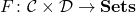be a functor, where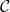is a filtered small category and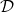is a finite category. Then the natural mapping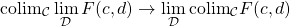is an isomorphism.

This statement is, for example, useful to check that a continuous morphism of sites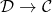commuting with finite limits induces a topos morphism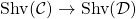, i.e. the pullback map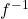is exact (note that a continuous site morphism does not induce a topos morphism in the general case).

The definition of a filtered system has a somewhat strange condition that it is non-empty. For some time I was not sure why it is essential to require this property. It turns out that without this condition, the filtered colimits will not commute with finite limits. Namely, the empty colimit will not commute with the empty limit (and only with it!).

I claim that the limit over an empty diagram in any category is simply a final object in that category.

Indeed, let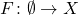be an empty diagram in a category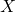. Then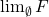is an object insuch that any other object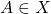admits exactly one morphism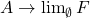. This means thatis a final object in. Clearly, a final object in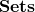is the one-point sets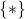.

Similarly, colimit on an empty diagram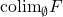is nothing more than an initial object in. Clearly, an initial object inis the empty set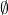.

Then we apply these considerations to the empty diagram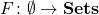, the natural map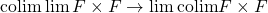is the map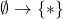that is clearly not an isomorphism!

Subscribe
Notify of
1 Comment
Inline Feedbacks